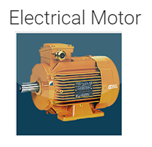# 7 – Best electric motor calculator apps for android and their features

1. Electric motorThis app will help us to wire or repair an electric motor and it also does electric motor calculations. This app also contains many motor wiring diagrams like rewinding wiring diagrams with description and motor symbols

By using this app we can do the motor speed calculation, number of pole calculations, and we can also calculate the frequency and horsepower of the motor. This app also has electrical calculator converters, like HP to KW converter, watts to joules, watts to KWH, KW to Kj, and Kw to KVA

2. Electric motor toolsBy using this app we can do many motor-related basic calculations like ampere, power for the single, and three-phase motor. This app will also help us to determine which horsepower rating motor has which NEMA frame size and by that, we can find the dimensions of the motor. It can also do certain functions like ampere and power calculation, motor mounting orientation and their designations, motor slip calc, Pulley calculations, poles vs RPM calculations

3. Simple motor calculator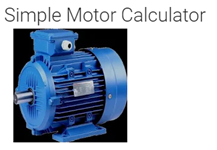The basic motor calculation can be done by using this app, it can calculate the induction motor starting component. By using this app we can also do other calculations such as the value of the motor current and capacity of the main star and delta contactors, overload, also circuit breaker.

4. Motor calculator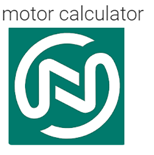This app will be really helpful for motor calculation, according to the input data we could get the desired calculations. This app also provides many schematic diagrams and it will be very useful. The input parameters could be voltage, power, power factor, efficiency, and start mode. We could find out the motor current, required capacitor, guard driver, etc.

5. Motor calculator pro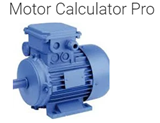By using this app we can do many motor calculations. In order to do the calculation click on the menu icon on the top left after installing the app, then pick the quantity that we need to do the calculations. While doing the calculations we could get two possibilities as results. With the help of this app, we can calculate the output power, efficiency, power factor, and lot more.

6. Motor calculator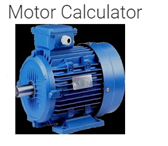This app will allow us to calculate the value and capacity of the contactor. We can also calculate the overload and circuit breaker and cable size by using this app. The input data that can be given to this app would be motor type, voltage, motor power, starting type. Then we could calculate the current value, overload range, main, star, and delta contactor capacity, circuit breaker range, overload range, etc.